GRE Subject Test: Math : Subsets

Example Questions

Example Question #1 : Real Analysis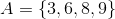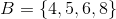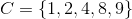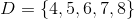Which of the following is true about the relationship between sets?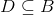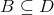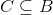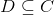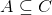Explanation:

The subset symbol should be read as "is a subset of" So it's the first letter is a subset of the second letter. To be a subset, all of its elements must be contained in the other set.

The only one of these relationships that is true (where the entire set on the left is in the right set) isThe elements of B, 4, 5, 6, and 8 all appear in set D.

Example Question #2 : Real Analysis

Which of the following is not a subset of Set A:{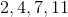}

{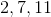}

{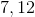}

{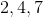}

{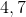}

{}

Explanation:

Step 1: A subset of a set must have elements in Set A. If any number in the subset is not in the original set, then that subset is not a subset of that set.

{} is not a subset becauseis not in Set A.

Example Question #3 : Real Analysis

Which of the following is NOT  a subset of Set A: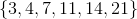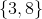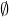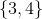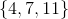Explanation:

Step 1: Recall the definition of a subset. A subset is a small part of a bigger given set; ALL numbers in the subset must be in the original set.

Step 2: We look at the original set and then look at the answers. If there is any answer that has an element that is not showing up in the original set, than that is the right answer.

Let's look at:. Theis in the original set, but theis not. Since we have a number in the subset that is not in the original set, we can say thatis not a subset.

The other two answers with brackets are subsets of the original set. All elements in the subsets are present in the original set.

The empty set is always a subset of any given set.

The answer isExample Question #4 : Real Analysis

What is a subset of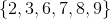?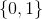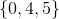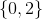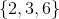Explanation:

Step 1: Define a subset. A subset of a bigger set is a smaller part where all the elements in the subset must be present in the bigger set.

The answer is. All numbers in this subset are in the bigger set, which is provided in the question...

Example Question #5 : Real Analysis

Which of the following is a subset of the set: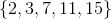?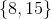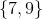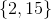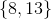Explanation:

Step 1: Define a subset

A subset of a big set is a set that has some of the elements that are in the bigger parent set.

The answer isbecause bothandare both in the bigger set.

All GRE Subject Test: Math Resources